# NCERT Solutions for Class 12 Physics Chapter 12 Atoms

In this chapter, we provide NCERT Solutions for Class 12 Physics Chapter 12 Atoms for English medium students, Which will very helpful for every student in their exams. Students can download the latest NCERT Solutions for Class 12 Physics Chapter 12 Atoms pdf, free NCERT solutions for Class 12 Physics Chapter 12 Atoms book pdf download. Now you will get step by step solution to each question. Class 12 physics is a very important subject for entrance exams like IIT JEE, CPMT, etc.

## NCERT Solutions for Class 12 Physics Chapter 12 Atoms

NCERT Exercises

Question 1.
Choose the correct alternative from the clues given at the end of each statement:
(a) The size of the atom in Thomson’s model is _____ the atomic size in Rutherford’s model. (Much greater than / no different from / much less than).
(b) In the ground state of _____ electrons are in stable equilibrium, while in _____ electrons always experience a net force. (Thomson’s model / Rutherford’s model).
(c) A classical atom based on _____ is doomed to collapse. (Thomson’s model / Rutherford’s model)
(d) An atom has a nearly continuous mass distribution in a _____ but has a highly non-uniform mass distribution in _____ (Thomson’s model / Rutherford’s model).
(e) The positively charged part of the atom possesses most of the mass in _____ (Rutherford’s model / both the models).
Solution:
(a) not different from
(b) Thomson’s model, Rutherford’s model
(c) Rutherford’s model
(d) Thomson’s model, Rutherford’s model
(e) both the models

Question 2.
Suppose you are given a chance to repeat the alpha-particle scattering experiment using a thin sheet of solid hydrogen in place of the gold foil. (Hydrogen is a solid at temperatures below 14 K). What results do you expect?
Solution:
The nucleus of a hydrogen atom is a proton. The mass of a proton is 1.67 × 10-27 kg, whereas the mass of an incident a-particle is 6.64 × 10-27 kg. Because the incident a-particles are more massive than the target nuclei (protons), the a-particle won’t bounce back even in a head on collision. It is similar to a football colliding with a tennis ball at rest. Thus, there would be no appreciable scattering.

Question 3.
What is the shortest wavelength present in the Paschen series of spectral lines?
Solution:
For shortest wavelength of Paschen series, n1 = 3, n2 = ∞Question 4.
A difference of 2.3 eV separates two energy levels in an atom. What is the frequency of radiation emitted when the atom makes a transition from the upper level to the lower level?
Solution:

Question 5.
The ground state energy of hydrogen atom is -13.6 eV. What are the kinetic and potential energies of the electron in this state?
Solution:

Question 6.
A hydrogen atom initially in the ground level absorbs a photon, which excites it to the n = 4 level. Determine the wavelength and frequency of photon.
Solution:
Energy of an electron in the nth orbit ofQuestion 7.
(a) Using the Bohr’s model, calculate the speed of the electron in a hydrogen atom in the n = 1, 2 and 3 levels,
(b) Calculate the orbital period in each of these levels.
Solution:
(a) Speed of the electron in Bohr’s nth orbit is(b) Orbital period of electron in Bohr’s first orbit is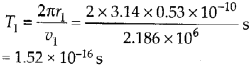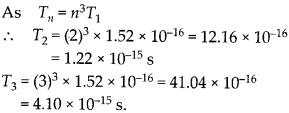Question 8.
The radius of the innermost electron orbit of a hydrogen atom is 5.3 × 10-11 m. What are the radii of the n = 2andn = 3 orbits?
Solution: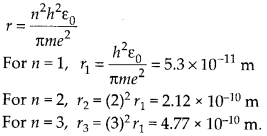Question 9.
A 12.5 eV electron beam is used to bombard gaseous hydrogen at room temperature. What series of wavelengths will be emitted?
Solution:
In ground state, energy of gaseous hydrogen at room temperature=-13.6 eV. When it is bombarded with 12.5 eV electron beam, the energy becomes – 13.6 + 12.5 = – 1.1 eV. The electron would jump from n = 1 to n = 3, 13 6 where E3 =$\cfrac { 13.6 }{ 3^{ 3 } }$ = -1.5 eV. On de-excitation the electron may jump from n = 3 to n = 2 giving rise to Balmer series. It may also jump from n = 3 to n = 1, giving rise to Lyman series.

Question 10.
ln accordance with the Bohr’s model, find the quantum number that characterises the earth’s revolution around the sun in an orbit of radius 1.5 × 1011 m with orbital speed 3 × 104 m s-1. (Mass of earth = 6.0 × 10224kg)
Solution:
According to Bohr’s quantization condition of angular momentum, Angular momentum of the earth around the sun,Question 11.
Answer the following questions, which helpyou understand the difference between Thomson’s model and Rutherford’s model better.
(a) Is the average angle of deflection of a-particles by a thin gold foil predicted by Thomson’s model much less, about the same, or much greater than that predicted by Rutherford’s model?
(b) Is the probability of backward scattering (i.e., scattering of a-particles at angles greater than 90°) predicted by Thomson’s model much less, about the same, or much greater than that predicted by Rutherford’s model?
(c) Keeping other factors fixed, it is found experimentally that for small thickness t, the number of a-particles scattered at moderate angles is proportional to What clue does this linear dependence on f provide?
(d) In which model is it completely wrong to ignore multiple scattering for the calculation of average angle of scattering of a-particles by a thin foil?
Solution:
(a) Nearly the same. This is because we are considering the average angle of deflection.
(b) Much less, because there is no such massive core (nucleus) in Thomson’s model as in Rutherford’s model.
(c) This suggests that scattering is mainly due to a single collision, because the chance of a single collision increases linearly with the number of the target atoms, and hence linearly with the thickness of the foil.
(d) In Thomson model, positive charge is distributed uniformly in the atom. So single collision causes very little deflection. The observed average scattering angle can be explained only by considering multiple scattering. Hence, it is wrong to ignore multiple scattering in Thomson’s model.

Question 12.
The gravitational attraction between electron and proton in a hydrogen atom is weaker than the coulomb attraction by a factor of about 10-40. An alternative way of looking at this fact is to estimate the radius of the first Bohr orbit of a hydrogen atom if the electron and proton were bound by gravitational attraction. You will find the answer interesting.
Solution:
The radius of the first orbit in Bohr’s model is calculated by considering the electrostatic force between electrons and proton in nucleus.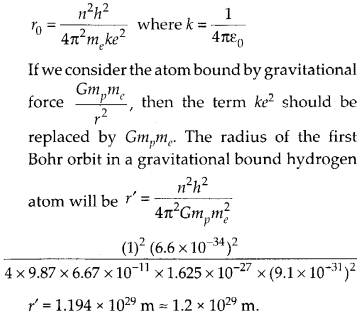This radius is much greater than the estimated size of the whole universe.

Question 13.
Obtain an expression for the frequency of radiation emitted when a hydrogen atom de-excites from level n to level (n – 1). For large n, show that this frequency equals the classical frequency of revolution of the electron in the orbit.
Solution:
Let us first find the frequency of revolution of electron in the orbit classically. In Bohr’s model velocity of electron in nthNow, let us find the frequency of radiation emitted when a hydrogen atom de-excites from level h to level (n – 1).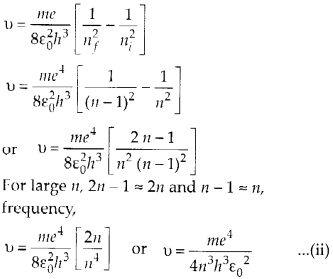Equation (i) and (ii) are equal, hence for large value of n, the classical frequency of revolution of electron in nth orbit is same as frequency of radiation when electron de-excite from level n to(n – l).

Question 14.
Classically, an electron can be in any orbit around the nucleus of an atom. Then what determines the typical atomic size? Why is an atom not, say, thousand times bigger than its typical size? The question had greatly puzzled Bohr before he arrived at his famous model of the atom that you have learnt in the text. To simulate what he might well have done before his discovery, let us play as follows with the basic constants of nature and see if we can get a quantity with the dimensions of length that is roughly equal to the known size of an atom 10 10 m)
(a) Construct a quantity with the dimensions of length from the fundamental constants e, me, and c. Determine its numerical value.
(b) You will find that the length obtained in (a) is many orders of magnitude smaller than the atomic dimensions. Further, it involves c. But energies of atoms are mostly in non-relativistic domain where c is not expected to play any role. This is what may have suggested Bohrto discard c and look for ‘something else’ to get the right atomic size. Now, the Planck’s constant h had already made its appearance elsewhere. Bohr’s great insight lay in recognising that h, me, and e will yield the right atomic size. Construct a quantity with the dimension of length from h, me, and e and confirm that its numerical value has indeed the correct order of magnitude.
Solution:(b) A quantity with the dimension of length from h, me and e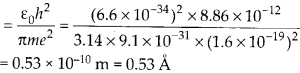The length is of the order of atomic size (10-10m)
Question 15.
The total energy of an electron in the first excited state of the hydrogen atom is about – 3.4 eV.
(a) What is the kinetic energy of the electron in this state?
(b) What is the potential energy of the electron in this state?
(c) Which of the answers above would change if the choice of the zero of potential energy is changed?
Solution:
Kinetic energy of an electron in an orbit,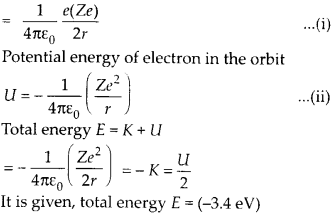(a) Kinetic energy of electron in this state E = -K
(b) Potential energy E = U/2, U = 2E = 2 (-3.4) = -6.8 eV
(c)
If thg zero of the potential energy is chosen differently, the kinetic energy remain the same. Although potential energy and hence total energy changes.

Question 16.
If Bohr’s quantisation postulate (angular momentum = nh/2n) is a basic law of nature, it should be equally valid for the case of planetary motion also. Why then do we never speak of quantisation of orbits of planets around the sun?
Solution:
Angular momentum mvr = n$\cfrac { h }{ 2\pi }$ associated with planetary motion are incomparably large relative to h. For example angular momentum of earth in its orbital motion is of the order of 1070$\cfrac { h }{ 2\pi }$.
For such large value of n, the difference in successive energies and angular momenta of the quantised levels of the Bohr model are so small that one can predict the energy level continuous.

Question 17.
Obtain the first Bohr’s radius and the ground state energy of a muonic hydrogen atom [/.e., an atom in which a negatively charged muon (μ) of mass about 207 me orbits around a proton].
Solution:
In Bohr’s model, the radius of nth orbit,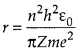In the given muonic hydrogen atom, a negatively charged muon (μ) of mass 207 me revolve around a proton. Therefore radius of electron and muon can be written asAll Chapter NCERT Solutions For Class12 Physics

—————————————————————————–

All Subject NCERT Solutions For Class12

*************************************************

Remark:

I think you got complete solutions for this chapter. If You have any queries regarding this chapter, please comment on the below section our subject teacher will answer you. We tried our best to give complete solutions so you got good marks in your exam.

If these solutions have helped you, you can also share Careerkundali.in to your friends.

Best of Luck!!